Remainder Theorem:

Remainder theorem states that when a polynomial function f(x) is divided by (x –a) where a is a constant, it will give us a remainder f(a).

Remainder of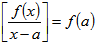Let us understand this with the help of some examples.

Example: Find the remainder of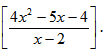Solution: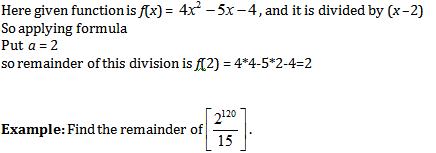Solution:

Method 1:

Here, note that numerator is in the form of powers of 2 hence we have to convert the denominator also in the form of powers of 2. Here denominator 15 = 24 –1

Now denominator is in the form of 24, so we can  write numerator also in the form of 24.

2120 =  (24)30. Now if take    24 = x

then numerator => x30

denominator = x –1.

Now by applying remainder theorem concept

a = 1 so remainder is f(a) =  (1)30 = 1.

Chinese Remainder Theorem:

Chinese theorem is used when we have a big composite number in denominator and complex power in numerator.

Let us understand this with the help of some examples:

Case 1: When remainder obtained by both numbers is equal

Example: Find the remainder of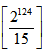Solution:

Step 1:

Write the denominator as product of two coprimes

In this case 15 = 5 * 3

And dedude remainder for respective factor

Step 2: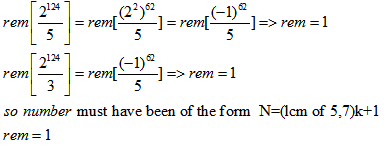Case 2: When remainders obtained by both numbers are unequal

Example: Find the remainder of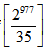Solution:

Step 1: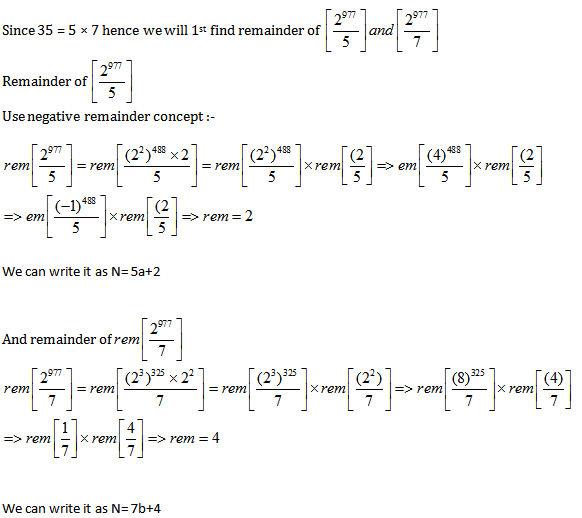Step 2:

Now we need values of a and b for that N is same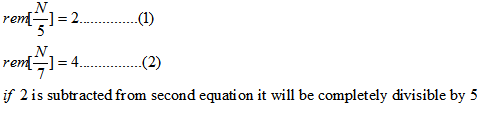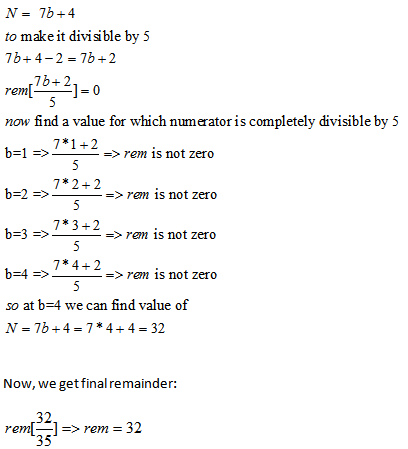Try out some more questions based on this concept so as to get a good hold of this topic.

EXERCISE:

Question 1. Find the remainder of .

(1) 22

(2) -22

(3) 11

(4) 0

Solution: Option 2

Here given function is f(x) = 3×3 –3x –4, and it is divided by (x + 2) hence here a = –2, so remainder of this division if f(–2) = 3 × (–2)3 –3 × (–2) –4 = –24 + 6–4 = –22

Question 2. Find remainder of .

(1) 12

(2) 32

(3) 162

(4) 0

Solution: Option 3

Step 1:

Split 100 into two coprimes

Since 100 = 25 × 4 hence we will 1st find remainder of

Step 2: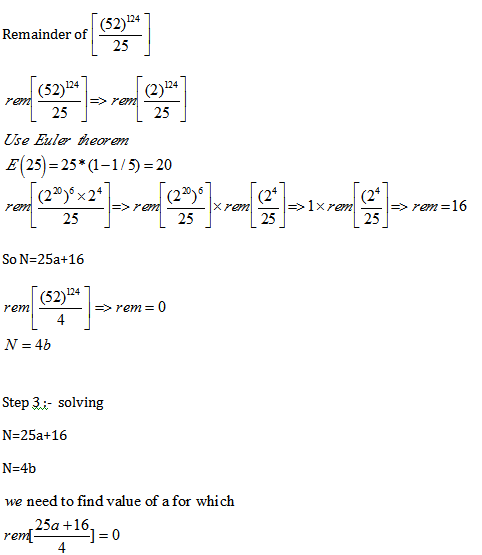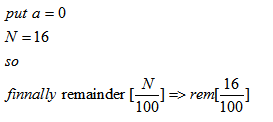Question 3. Find remainder of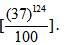(1) 37

(2) 52

(3) 16

(4) 61

Solution: Option 4

Step 1 :

Split 100 into two coprimes

Since 100 = 25 × 4 hence we will 1st find remainder of

Step 2:-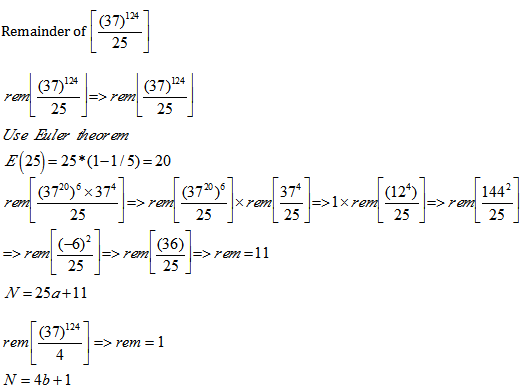Step 3 :

We need to find value of a for that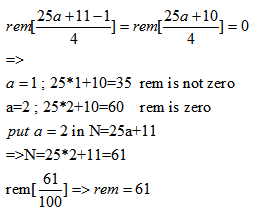Get Posts Like This Sent to your Email
Updates for Free Live sessions and offers are sent on mail. Don't worry: we do not send too many emails..:)
Get Posts Like This Sent to your Email
Updates for Free Live sessions and offers are sent on mail. Don't worry: we do not send too many emails..:)Get the latest updates from our side, including offers and free live updates, on email.Leverage agile frameworks to provide a robust synopsis for high level overviews.
Special Launch Offer: 20% off all courses till 24th Jan, 2023.
10
days
10
hours
10
minutes
10
seconds
4 Short Term Courses launched for CAT-2021. Special 10% off as launch offer.
10
days
10
hours
10
minutes
10
seconds